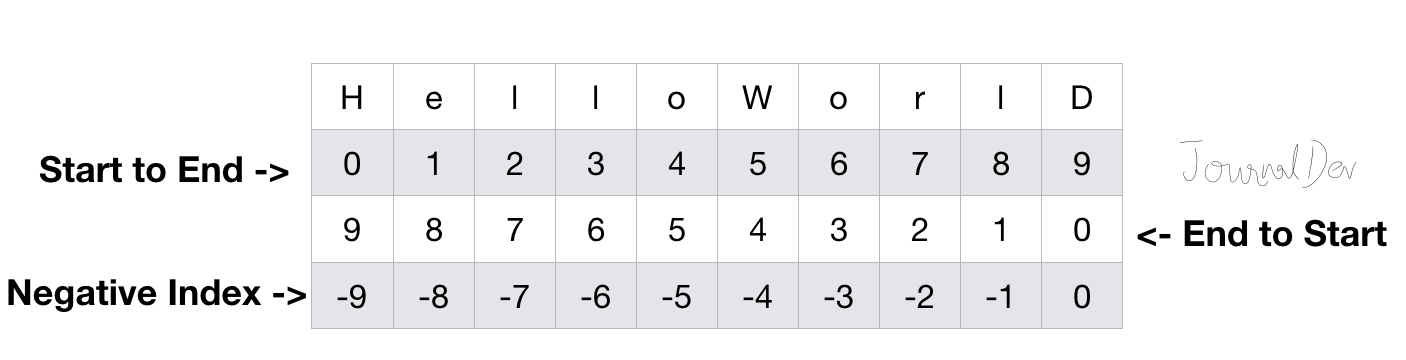# Python slice string

Published on August 3, 2022By Pankaj
Developer and author at DigitalOcean.While we believe that this content benefits our community, we have not yet thoroughly reviewed it. If you have any suggestions for improvements, please let us know by clicking the “report an issue“ button at the bottom of the tutorial.

Python string supports slicing to create substring. Note that Python string is immutable, slicing creates a new substring from the source string and original string remains unchanged.

## Python slice string

Python slice string syntax is:

``````str_object[start_pos:end_pos:step]
``````

The slicing starts with the start_pos index (included) and ends at end_pos index (excluded). The step parameter is used to specify the steps to take from start to end index. Python String slicing always follows this rule: s[:i] + s[i:] == s for any index ‘i’. All these parameters are optional - start_pos default value is 0, the end_pos default value is the length of string and step default value is 1. Let’s look at some simple examples of string slice function to create substring.

``````s = 'HelloWorld'

print(s[:])

print(s[::])
``````

Output:

``````HelloWorld
HelloWorld
``````

Note that since none of the slicing parameters were provided, the substring is equal to the original string. Let’s look at some more examples of slicing a string.

``````s = 'HelloWorld'
first_five_chars = s[:5]
print(first_five_chars)

third_to_fifth_chars = s[2:5]
print(third_to_fifth_chars)
``````

Output:

``````Hello
llo
``````

Note that index value starts from 0, so start_pos 2 refers to the third character in the string.

### Reverse a String using Slicing

We can reverse a string using slicing by providing the step value as -1.

``````s = 'HelloWorld'
reverse_str = s[::-1]
print(reverse_str)
``````

Output: `dlroWolleH` Let’s look at some other examples of using steps and negative index values.

``````s1 = s[2:8:2]
print(s1)
``````

Output: `loo` Here the substring contains characters from indexes 2,4 and 6.

``````s1 = s[8:1:-1]
print(s1)
``````

Output: `lroWoll` Here the index values are taken from end to start. The substring is made from indexes 1 to 7 from end to start.``````s1 = s[8:1:-2]
print(s1)
``````

Output: `lool`Python slice works with negative indexes too, in that case, the start_pos is excluded and end_pos is included in the substring.

``````s1 = s[-4:-2]
print(s1)
``````

Output: `or`Python string slicing handles out of range indexes gracefully.

``````>>>s = 'Python'
>>>s[100:]
''
>>>s[2:50]
'thon'
``````

That’s all for python string slice function to create substring.

You can checkout complete python script and more Python examples from our GitHub Repository.Pankaj

author

Developer and author at DigitalOcean.

#### Still looking for an answer?

Plz tell me the slice of ‘HeloWr’

TheData = [20, 3, 4,8,12, 99,4, 26 , 4 ] TheData1= TheData [ 0 : len(TheData) : 1 ] def InsertionData (TheData1): for Count in range(0, len(TheData1)): DataToInsert = TheData1(Count) Inserted = 0 Nextvalue = Count - 1 while (Nextvalue >= 0 and Inserted != 1): if DataToInsert < TheData1(Nextvalue): TheData1(Nextvalue + 1) == TheData1(Nextvalue) Nextvalue = Nextvalue -1 TheData1(Nextvalue +1 ) == DataToInsert else: Inserted = 1 def printarray(TheData1): for count in range(0, len(TheData1)): print(TheData1(count) ) print("Array before sorting \n ") printarray(TheData1) InsertionData(TheData1) print(“Array After sorting \n”) printarray(TheData1) Error: Array before sorting Traceback (most recent call last): File “c:\Users\Admin\Desktop\Paper 4 solution.py”, line 26, in printarray(TheData1) File “c:\Users\Admin\Desktop\Paper 4 solution.py”, line 23, in printarray print(TheData1(count) ) TypeError: ‘list’ object is not callable Anyone help how to done slicing in python

- malik

what does s(5:5) return

Your idea about reverse a string using a negative value is completely wrong please update this post. What actually happening here is x = ‘H e l l o w o r l d’ -----0 1 2 3 4 5 6 7 8 9 >>> x[8:1:-1] output – ‘ l r o w o l l ‘ -------8 7 6 5 4 3 2 Usually, the second parameter 1 won’t be taken into consideration right. This means if I enter x[1:8], index 8 won’t be sliced right. Similarly, this happens in x[8:1:-1]. So index 1 won’t be sliced. >>> x[-2:-8:-1] output- ‘lrowol’ Here, -2,-3,-4,-5,-6,-7 indexes will be sliced. Conclusion: string[x:y:p] When p x>y and index y won’t be sliced. That’ it!!!

- Kobinarth Panchalingam

- mostafa

For the negative count, the end of the string starts from -1 not 0. It is like -4 -3 -2 -1 not -4 -3 -2 -1 0

- Coder

Hi I have a doubt: string = “Hi There” print (string[-4:-2]) and the output is ‘he’ but shouldn’t the output be ‘eh’? I am unable to understand why the output is showing he

- Kalpit

Can you explain the last one a little bit? s[-4:-2] Thanks!

- Enoc

Hi Thankx for this section. I need more clarification on s1 = s[8:1:-1] print(s1) Output: lroWoll I am unable to get it .

thanks pankaj!!!

- curry lover 68